xWettingEncyclopedia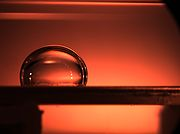>

Wetting is the ability of a liquid
Liquid
Liquid is one of the three classical states of matter . Like a gas, a liquid is able to flow and take the shape of a container. Some liquids resist compression, while others can be compressed. Unlike a gas, a liquid does not disperse to fill every space of a container, and maintains a fairly...

to maintain contact with a solid
Solid
Solid is one of the three classical states of matter . It is characterized by structural rigidity and resistance to changes of shape or volume. Unlike a liquid, a solid object does not flow to take on the shape of its container, nor does it expand to fill the entire volume available to it like a...

surface
Surface
In mathematics, specifically in topology, a surface is a two-dimensional topological manifold. The most familiar examples are those that arise as the boundaries of solid objects in ordinary three-dimensional Euclidean space R3 — for example, the surface of a ball...

, resulting from intermolecular interactions when the two are brought together. The degree of wetting (wettability) is determined by a force balance between adhesive
Adhesion is any attraction process between dissimilar molecular species that can potentially bring them in close contact. By contrast, cohesion takes place between similar molecules....

and cohesive
Cohesion (chemistry)
Cohesion or cohesive attraction or cohesive force is the action or property of like molecules sticking together, being mutually attractive...

force
Force
In physics, a force is any influence that causes an object to undergo a change in speed, a change in direction, or a change in shape. In other words, a force is that which can cause an object with mass to change its velocity , i.e., to accelerate, or which can cause a flexible object to deform...

s.

Wetting is important in the bonding
Chemical bond
A chemical bond is an attraction between atoms that allows the formation of chemical substances that contain two or more atoms. The bond is caused by the electromagnetic force attraction between opposite charges, either between electrons and nuclei, or as the result of a dipole attraction...

Adhesion is any attraction process between dissimilar molecular species that can potentially bring them in close contact. By contrast, cohesion takes place between similar molecules....

of two materials. Wetting and the surface forces that control wetting are also responsible for other related effects, including so-called capillary
Capillary action
Capillary action, or capilarity, is the ability of a liquid to flow against gravity where liquid spontanously rise in a narrow space such as between the hair of a paint-brush, in a thin tube, or in porous material such as paper or in some non-porous material such as liquified carbon fiber, or in a...

effects. Regardless of the amount of wetting, the shape of a liquid drop on a rigid surface is roughly a truncated sphere
Spherical cap
In geometry, a spherical cap is a portion of a sphere cut off by a plane. If the plane passes through the center of the sphere, so that the height of the cap is equal to the radius of the sphere, the spherical cap is called a hemisphere....

## Explanation

An adhesive, or glue, is a mixture in a liquid or semi-liquid state that adheres or bonds items together. Adhesives may come from either natural or synthetic sources. The types of materials that can be bonded are vast but they are especially useful for bonding thin materials...

forces between a liquid
Liquid
Liquid is one of the three classical states of matter . Like a gas, a liquid is able to flow and take the shape of a container. Some liquids resist compression, while others can be compressed. Unlike a gas, a liquid does not disperse to fill every space of a container, and maintains a fairly...

and solid
Solid
Solid is one of the three classical states of matter . It is characterized by structural rigidity and resistance to changes of shape or volume. Unlike a liquid, a solid object does not flow to take on the shape of its container, nor does it expand to fill the entire volume available to it like a...

cause a liquid drop to spread across the surface
Surface
In mathematics, specifically in topology, a surface is a two-dimensional topological manifold. The most familiar examples are those that arise as the boundaries of solid objects in ordinary three-dimensional Euclidean space R3 — for example, the surface of a ball...

. Cohesive
Cohesion (chemistry)
Cohesion or cohesive attraction or cohesive force is the action or property of like molecules sticking together, being mutually attractive...

forces within the liquid cause the drop to ball up and avoid contact with the surface.

wetting
! colspan=2 |Strength of:
|-
! Sol./Liq.
interactions
! Liq./Liq.
interactions
|-
| θ = 0 ||Perfect wetting ||strong||weak
|-
| rowspan=2 |0 < θ < 90°
| rowspan=2 |high wettability
| strong ||strong
|-
| weak || weak
|-
| 90° ≤ θ < 180°||low wettability ||weak||strong
|-
| θ = 180°||perfectly
non-wetting ||weak||strong
|}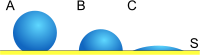The contact angle
Contact angle
The contact angle is the angle at which a liquid/vapor interface meets a solid surface. The contact angle is specific for any given system and is determined by the interactions across the three interfaces. Most often the concept is illustrated with a small liquid droplet resting on a flat...

(θ), as seen in Figure 1, is the angle at which the liquid–vapor interface meets the solid–liquid interface. The contact angle is determined by the resultant between adhesive and cohesive forces. As the tendency of a drop to spread out over a flat, solid surface increases, the contact angle decreases. Thus, the contact angle provides an inverse measure of wettability.

A contact angle less than 90° (low contact angle) usually indicates that wetting of the surface is very favorable, and the fluid will spread over a large area of the surface. Contact angles greater than 90° (high contact angle) generally means that wetting of the surface is unfavorable so the fluid will minimize contact with the surface and form a compact liquid droplet.

For water
Water
Water is a chemical substance with the chemical formula H2O. A water molecule contains one oxygen and two hydrogen atoms connected by covalent bonds. Water is a liquid at ambient conditions, but it often co-exists on Earth with its solid state, ice, and gaseous state . Water also exists in a...

, a wettable surface may also be termed hydrophilic and a non-wettable surface hydrophobic. Superhydrophobic surfaces have contact angles greater than 150°, showing almost no contact between the liquid drop and the surface. This is sometimes referred to as the "Lotus effect
Lotus effect
The lotus effect refers to the very high water repellence exhibited by the leaves of the lotus flower ....

". The table describes varying contact angles and their corresponding solid/liquid and liquid/liquid interactions. For non-water liquids, the term lyophilic is used for low contact angle conditions and lyophobic is used when higher contact angles result. Similarly, the terms omniphobic and omniphilic apply to both polar and apolar liquids.

## High energy vs. low energy surfaces

There are two main types of solid surfaces with which liquids can interact. Traditionally, solid surfaces have been divided into high energy
Energy
In physics, energy is an indirectly observed quantity. It is often understood as the ability a physical system has to do work on other physical systems...

solids and low energy types. The relative energy of a solid has to do with the bulk nature of the solid itself. Solids such as metals, glass
Glass
Glass is an amorphous solid material. Glasses are typically brittle and optically transparent.The most familiar type of glass, used for centuries in windows and drinking vessels, is soda-lime glass, composed of about 75% silica plus Na2O, CaO, and several minor additives...

es, and ceramic
Ceramic
A ceramic is an inorganic, nonmetallic solid prepared by the action of heat and subsequent cooling. Ceramic materials may have a crystalline or partly crystalline structure, or may be amorphous...

s are known as 'hard solids' because the chemical bond
Chemical bond
A chemical bond is an attraction between atoms that allows the formation of chemical substances that contain two or more atoms. The bond is caused by the electromagnetic force attraction between opposite charges, either between electrons and nuclei, or as the result of a dipole attraction...

s that hold them together (e.g., covalent, ionic, or metallic) are very strong. Thus, it takes a large input of energy to break these solids so they are termed “high energy.” Most molecular liquids achieve complete wetting with high-energy surfaces.

The other type of solids is weak molecular crystals (e.g., fluorocarbons, hydrocarbons, etc.) where the molecules are held together essentially by physical forces (e.g., van der Waals and hydrogen bonds). Since these solids are held together by weak forces it would take a very low input of energy to break them, and thus, they are termed “low energy.” Depending on the type of liquid chosen, low-energy surfaces can permit either complete or partial wetting.

### Wetting of low energy surfaces

Low-energy surfaces primarily interact with liquids through dispersion (van der Waals
Van der Waals force
In physical chemistry, the van der Waals force , named after Dutch scientist Johannes Diderik van der Waals, is the sum of the attractive or repulsive forces between molecules other than those due to covalent bonds or to the electrostatic interaction of ions with one another or with neutral...

) forces. William Zisman
William Zisman
Dr. William Albert Zisman was an American chemist and geophysicist.He was born in Albany, NY and spent his youth in Providence, RI up to the age of 14 when his family moved to Washington, DC. He earned his BS and MS degrees in physics from Massachusetts Institute of Technology. He began his career...

had several key findings in the work that he did:
• Zisman observed that cos θ increases linearly as the surface tension
Surface tension
Surface tension is a property of the surface of a liquid that allows it to resist an external force. It is revealed, for example, in floating of some objects on the surface of water, even though they are denser than water, and in the ability of some insects to run on the water surface...

(γLV) of the liquid decreased. Thus, he was able to establish a rectilinear
Rectilinear
Rectilinear may refer to:* Rectilinear grid, a tessellation of the Euclidean plane* Rectilinear lens, a photographic lens* Rectilinear locomotion, a form of animal locomotion* Rectilinear polygon, a polygon whose edges meet at right angles...

relation between cos θ and the surface tension (γLV) for various organic
Organic compound
An organic compound is any member of a large class of gaseous, liquid, or solid chemical compounds whose molecules contain carbon. For historical reasons discussed below, a few types of carbon-containing compounds such as carbides, carbonates, simple oxides of carbon, and cyanides, as well as the...

liquids.

A surface is more wettable when γLV is low and when θ is low. He termed the intercept of these lines when the cos θ = 1, as the critical surface tension (γc) of that surface. This critical surface tension is an important parameter because it is a characteristic of only the solid.

Knowing the critical surface tension of a solid, it is possible to predict the wettability of the surface.
• The wettability of a surface is determined by the outermost chemical groups of the solid.
• Differences in wettability between surfaces that are similar in structure are due to differences in packing of the atoms. For instance, if a surface has branched chains, it will have poorer packing than a surface with straight chains.

## Ideal solid surfaces

An ideal solid surface is one that is flat, rigid, perfectly smooth, chemically homogeneous, and has zero contact angle hysteresis. Zero hysteresis
Hysteresis
Hysteresis is the dependence of a system not just on its current environment but also on its past. This dependence arises because the system can be in more than one internal state. To predict its future evolution, either its internal state or its history must be known. If a given input alternately...

implies that the advancing and receding contact angles are equal. In other words, there is only one thermodynamically stable contact angle. When a drop of liquid is placed on such a surface, the characteristic contact angle is formed as depicted in Fig. 1. Furthermore, on an ideal surface the drop will return to its original shape if it is disturbed. The following derivations apply only to ideal solid surfaces. In other words, they are only valid for the state in which the interfaces are not moving and the phase boundary line exists in equilibrium.

### Minimization of energy, three phases

Figure 3 shows the line of contact where three phases meet. In equilibrium
Thermodynamic equilibrium
In thermodynamics, a thermodynamic system is said to be in thermodynamic equilibrium when it is in thermal equilibrium, mechanical equilibrium, radiative equilibrium, and chemical equilibrium. The word equilibrium means a state of balance...

, the net force
Force
In physics, a force is any influence that causes an object to undergo a change in speed, a change in direction, or a change in shape. In other words, a force is that which can cause an object with mass to change its velocity , i.e., to accelerate, or which can cause a flexible object to deform...

per unit length acting along the boundary line between the three phases must be zero. The components of net force in the direction along each of the interfaces are given by: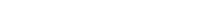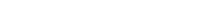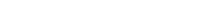where α, β, and θ are the angles shown and γij is the surface energy between the two indicated phases. These relations can also be expressed by an analog to a triangle known as Neumann’s triangle, shown in Figure 4. Neumann’s triangle is consistent with the geometrical restriction that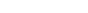, and applying the law of sines and law of cosines to it produce relations that describe how the interfacial angles depend on the ratios of surface energies.

Because these three surface energies form the sides of a triangle
Triangle
A triangle is one of the basic shapes of geometry: a polygon with three corners or vertices and three sides or edges which are line segments. A triangle with vertices A, B, and C is denoted ....

, they are constrained by the triangle inequalities, γij < γjk + γik meaning that no one of the surface tensions can exceed the sum of the other two. If three fluids with surface energies that do not follow these inequalities are brought into contact, no equilibrium configuration consistent with Figure 3 will exist.

#### Simplification to planar geometry, Young's relation

If the β phase is replaced by a flat rigid surface, as shown in Figure 5, then β = π, and the second net force equation simplifies to the Young equation,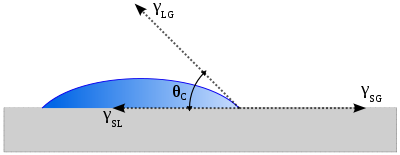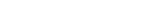which relates the surface tensions between the three phases: solid
Solid
Solid is one of the three classical states of matter . It is characterized by structural rigidity and resistance to changes of shape or volume. Unlike a liquid, a solid object does not flow to take on the shape of its container, nor does it expand to fill the entire volume available to it like a...

, liquid
Liquid
Liquid is one of the three classical states of matter . Like a gas, a liquid is able to flow and take the shape of a container. Some liquids resist compression, while others can be compressed. Unlike a gas, a liquid does not disperse to fill every space of a container, and maintains a fairly...

and gas
Gas
Gas is one of the three classical states of matter . Near absolute zero, a substance exists as a solid. As heat is added to this substance it melts into a liquid at its melting point , boils into a gas at its boiling point, and if heated high enough would enter a plasma state in which the electrons...

. Subsequently this predicts the contact angle of a liquid droplet on a solid surface from knowledge of the three surface energies involved. This equation also applies if the "gas" phase is another liquid, immiscible with the droplet of the first "liquid" phase.

#### Real Smooth Surfaces and the Young contact angle

The Young equation mentioned above assumes a perfectly flat and rigid surface. In many cases surface are far from this ideal situation, and there are two cases that we consider here. The case of rough surfaces is discussed in the paragraph below (see Non-ideal rough solid surfaces), and the case of smooth surfaces that are still real (finitely rigid) is discussed here. Even in a perfectly smooth surface a drop will assume a wide spectrum of contact angles ranging from the so called advancing contact angle,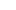, to the so called receding contact angle,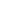. The equilibrium contact angle (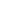) can be calculated fromandas was shown by Tadmor as,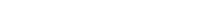where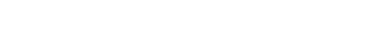#### The Young–Dupré equation and Spreading Coefficient

The Young–Dupré equation (Thomas Young 1805, Lewis Dupré 1855) dictates that neither γSG nor γSL can be larger than the sum of the other two surface energies. The consequence of this restriction is the prediction of complete wetting when γSG > γSL + γLG and zero wetting when γSL > γSG + γLG. The lack of a solution to the Young–Dupré equation is an indicator that there is no equilibrium configuration with a contact angle between 0 and 180° for those situations.

A useful parameter for gauging wetting is the spreading parameter S,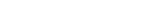When S > 0, the liquid wets the surface completely (complete wetting).
When S < 0, there is partial wetting.

Combining the spreading parameter definition with the Young relation, we obtain the Young–Dupré equation: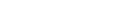which only has physical solutions for θ when S < 0.

## Non-ideal rough solid surfaces

Unlike ideal surfaces, real surfaces do not have perfect smoothness, rigidity, or chemical homogeneity. Such deviations from ideality result in phenomena called contact-angle hysteresis. Contact-angle hysteresis is defined as the difference between the advancing (θa) and receding (θr) contact angles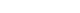In simpler terms, contact angle hysteresis is essentially the displacement of a contact line such as the one in figure 3, by either expansion or retraction of the droplet. Figure 6 depicts the advancing and receding contact angles. The advancing contact angle is the maximum stable angle, whereas the receding contact angle is the minimum stable angle. Contact-angle hysteresis occurs because there are many different thermodynamically stable contact angles on a non-ideal solid. These varying thermodynamically stable contact angles are known as metastable states.

Such motion of a phase boundary, involving advancing and receding contact angles, is known as dynamic wetting. When a contact line advances, covering more of the surface with liquid, the contact angle is increased and generally is related to the velocity of the contact line. If the velocity of a contact line is increased without bound, the contact angle increases, and as it approaches 180° the gas phase will become entrained in a thin layer between the liquid and solid. This is a kinetic non-equilibrium effect which results from the contact line moving at such a high speed that complete wetting cannot occur.

A well known departure from ideality is when the surface of interest has a rough texture. The rough texture of a surface can fall into one of two categories: homogeneous or heterogeneous. A homogeneous wetting regime is where the liquid fills in the roughness grooves of a surface. On the other hand, a heterogeneous wetting regime is where the surface is a composite of two types of patches. An important example of such a composite surface is one composed of patches of both air and solid. Such surfaces have varied effects on the contact angles of wetting liquids. Cassie–Baxter and Wenzel are the two main models that attempt describe the wetting of textured surfaces. However, these equations only apply when the drop size is sufficiently large compared with the surface roughness scale.

### Wenzel's model

The Wenzel model (Robert N. Wenzel 1936) describes the homogeneous wetting regime, as seen in Figure 7, and is defined by the following equation for the contact angle on a rough surface: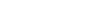where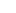is the apparent contact angle which corresponds to the stable equilibrium state (i.e. minimum free energy state for the system). The roughness ratio, r, is a measure of how surface roughness affects a homogeneous surface. The roughness ratio is defined as the ratio of true area of the solid surface to the apparent area.

θ is the Young contact angle as defined for an ideal surface. Although Wenzel's equation demonstrates that the contact angle of a rough surface is different from the intrinsic contact angle, it does not describe contact angle hysteresis.

### Cassie–Baxter model

When dealing with a heterogeneous surface, the Wenzel model is not sufficient. A more complex model is needed to measure how the apparent contact angle changes when various materials are involved. This heterogeneous surface, like that seen in Figure 8, is explained using the Cassie–Baxter equation (Cassie's law
Cassie's law
Cassie's law describes the effective contact angle θc for a liquid on a composite surface . The law explains how simply roughing up a surface increases the apparent surface angle...

):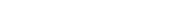Here the rf is the roughness ratio of the wet surface area and f is the fraction of solid surface area wet by the liquid. It is important to realize that when f = 1 and rf = r, the Cassie–Baxter equations becomes the Wenzel equation. On the other hand, when there are many different fractions of surface roughness, each fraction of the total surface area is denoted by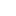.

A summation of all fi equals 1 or the total surface. Cassie–Baxter can also be recast in the following equation: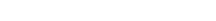Here γ is the Cassie–Baxter surface tension and between liquid and vapor, the γi,sv is the solid vapor surface tension of every component and γi,sl is the solid liquid surface tension of every component. A case that is worth mentioning is when the liquid drop is placed on the substrate and creates small air pockets underneath it. This case for a two component system is denoted by:Here the key difference to notice is that the there is no surface tension between the solid and the vapor for the second surface tension component. This is because we assume that the surface of air that is exposed is under the droplet and is the only other substrate in the system. Subsequently the equation is then expressed as (1 – f). Therefore the Cassie equation can be easily derived from the Cassie–Baxter equation. Experimental results regarding the surface properties of Wenzel versus Cassie–Baxter systems showed the effect of pinning for a Young angle of 180° to 90°, a region classified under the Cassie–Baxter model. This liquid air composite system is largely hydrophobic. After that point a sharp transition to the Wenzel regime was found where the drop wets the surface but no further than edges of the drop.

#### Precursor film

With the advent of high resolution imaging, researchers have started to obtain experimental data which has led them to question the assumptions of the Cassie–Baxter equation when calculating the apparent contact angle. These groups believe that the apparent contact angle is largely dependent on the triple line. The triple line, which is in contact with the heterogeneous surface, cannot rest on the heterogeneous surface like the rest of the drop. In theory it should follow the surface imperfection. This bending in triple line is unfavorable and is not seen in real world situations. A theory that preserves the Cassie–Baxter equation while at the same time explaining the presence of minimized energy state of the triple line hinges on the idea of a precursor film. This film of submicrometer thickness advances ahead of the motion of the droplet and is found around the triple line. Furthermore, this precursor film allows the triple line to bend and take different conformations that were originally considered unfavorable. This precursor fluid has been observed using environmental scanning electron microscopy (ESEM) in surfaces with pores formed in the bulk. With the introduction of the precursor film concept, the triple line can follow energetically feasible conformations and thereby correctly explaining the Cassie–Baxter model.

#### "Petal effect" vs. "lotus effect"

The intrinsic hydrophobicity of a surface can be enhanced by being textured with different length scales of roughness. The red rose takes advantage of this by using a hierarchy of micro- and nanostructures on each petal to provide sufficient roughness for superhydrophobicity. More specifically, each rose petal has a collection of micropapillae on the surface and each papillae, in turn, has many nanofolds. The term “petal effect” describes the fact that a water droplet on the surface of a rose petal is spherical in shape, but cannot roll off even if the petal is turned upside down. The water drops maintain their spherical shape due to the superhydrophobicity of the petal (contact angle of about 152.4°), but do not roll off because the petal surface has a high adhesive force with water.

When comparing the "petal effect" to the "lotus effect
Lotus effect
The lotus effect refers to the very high water repellence exhibited by the leaves of the lotus flower ....

", it is important to note some striking differences. The surface structure of the lotus petal and the rose petal, as seen in Figure 9, can be used to explain the two different effects. The lotus petal has a randomly rough surface and low contact angle hysteresis, which means that the water droplet is not able to wet the microstructure spaces between the spikes. This allows air to remain inside the texture, causing a heterogeneous surface composed of both air and solid. As a result, the adhesive force between the water and the solid surface is extremely low, allowing the water to roll off easily (i.e. "self-cleaning" phenomena).

On the other hand, the rose petal's micro- and nanostructures are larger in scale than the lotus leaf, which allows the liquid film to impregnate the texture. However, as seen in Figure 9, the liquid can enter the larger scale grooves, but it cannot enter into the smaller grooves. This is known as the Cassie impregnating wetting regime. Since the liquid can wet the larger scale grooves, the adhesive force between the water and solid is very high. This explains why the water droplet will not fall off even if the petal is tilted at an angle or turned upside down. However, this effect will fail if the droplet has a volume larger than 10 µL because the balance between weight and surface tension is surpassed.

### Cassie–Baxter to Wenzel transition

In the Cassie–Baxter model
Cassie's law
Cassie's law describes the effective contact angle θc for a liquid on a composite surface . The law explains how simply roughing up a surface increases the apparent surface angle...

, the drop sits on top of the textured surface with trapped air underneath. During the wetting transition
Wetting transition
-Wetting transitions:The macroscopic parameter characterizing wetting of a solid surface with a liquid is contact angle. Various contact angles can co-exist on the same solid substrate. When contact angle experiences change we observe a wetting transition. Wetting transitions occurring on flat and...

from the Cassie state to the Wenzel state, the air pockets are no longer thermodynamically stable and liquid begins to nucleate from the middle of the drop, creating a “mushroom state,” as seen in Figure 10. The penetration condition is given by the following equation: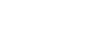where
• θC is the critical contact angle
• φ is the fraction of solid/liquid interface where drop is in contact with surface
• r is solid roughness (for flat surface, r = 1)

The penetration front propagates to minimize the surface energy until it reaches the edges of the drop, thus arriving at the Wenzel state. Since the solid can be considered an absorptive material due to its surface roughness, this phenomenon of spreading and imbibition is called hemi-wicking. The contact angles at which spreading/imbibition occurs are between 0 ≤ θ < π/2.

The Wenzel model is valid between θC < θ < π/2. If the contact angle is less than ΘC, the penetration front spreads beyond the drop and a liquid film forms over the surface. Figure 11 depicts the transition from the Wenzel state to the surface film state. The film smoothes the surface roughness and the Wenzel model no longer applies. In this state, the equilibrium condition and Young's relation yields: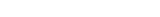By fine tuning the surface roughness, it is possible achieves a transition between both super hydrophobic and super hydrophilic regions. Generally, the rougher the surface, the more hydrophobic it is.

## Effect of surfactants on wetting

Many technological processes require control of liquid spreading over solid surfaces. When a drop is placed on a surface, it can completely wet, partially wet, or not wet the surface. By reducing the surface tension with surfactants, a non-wetting material can be made to become partially or completely wetting. The excess free energy (σ) of a drop on a solid surface is: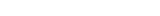• γ is the liquid–vapor interfacial tension
• γSL is the solid–liquid interfacial tension
• γSV is the solid–vapor interfacial tension
• S is the area of liquid–vapor interface
• P is the excess pressure inside liquid
• R is the radius of droplet base

Based on this equation, the excess free energy is minimized when γ decreases, γSL decreases, or γSV increases. Surfactants are absorbed onto the liquid–vapor, solid–liquid, and solid–vapor interfaces, which modify the wetting behavior of hydrophobic materials to reduce the free energy. When surfactants are absorbed onto a hydrophobic surface, the polar head groups face into the solution with the tail pointing outward. In more hydrophobic surfaces, surfactants may form a bilayer on the solid, causing it to become more hydrophilic. The dynamic drop radius can be characterized as the drop begins to spread. Thus, the contact angle changes based on the following equation: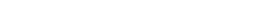• θ0 is initial contact angle
• θ is final contact angle
• τ is the surfactant transfer time scale

As the surfactants are absorbed, the solid–vapor surface tension increases and the edges of the drop become hydrophilic. As a result, the drop spreads.

Adsorption is the adhesion of atoms, ions, biomolecules or molecules of gas, liquid, or dissolved solids to a surface. This process creates a film of the adsorbate on the surface of the adsorbent. It differs from absorption, in which a fluid permeates or is dissolved by a liquid or solid...

• Amott index
Amott index
The Amott test is one of the most widely used empirical wettability measurements for reservoir cores in petroleum engineering. The method combines two spontaneous imbibition measurements and two forced displacement measurements...

• Anti-fog
Anti-fog
Anti-fog agents, also known as anti-fogging agents and treatments, are chemicals which prevent the condensation of water in the form of small droplets on a surface which resemble fog...

• Dewetting
Dewetting
In fluid mechanics, dewetting is one of the processes that can occur at a solid–liquid or liquid–liquid interface. Generally, dewetting describes the rupture of a thin liquid film on the substrate and the formation of droplets. The opposite process—spreading of a liquid on a substrate—is called...

• Electrowetting
Electrowetting
Electrowetting is the modification of the wetting properties of a surface with an applied electric field.-History:...

• Flotation
Buoyancy
In physics, buoyancy is a force exerted by a fluid that opposes an object's weight. In a column of fluid, pressure increases with depth as a result of the weight of the overlying fluid. Thus a column of fluid, or an object submerged in the fluid, experiences greater pressure at the bottom of the...

• Sessile drop technique